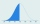# Comparing + probability - math problems

#### Number of problems found: 5

• Suppose 4Suppose that 14% of all steel shafts produced by a certain process are nonconforming but can be reworked (rather than having to be scrapped). Consider a random sample of 200 shafts, and let X denote the number among these that are nonconforming and can be
• SeedsFrom a box of spruce seeds with the germination of 80%, we randomly select 10 seeds and plant them. Find the median of the random variable: the number of germinating seeds.
• A mountain climberA mountain climber plans to buy some rope to use as lifeline. Which of the following would be the better choice? Explain your choice. Rope A: Mean breaking strength:500lb; standard deviation of 100lb Rope B: Mean breaking strength: 500lb; standard deviati
• Perfect cubesSuppose a number is chosen at random from the set (0,1,2,3,. .. ,202). What is the probability that the number is a perfect cube?
• Sum on diceWe have two dice. What is the greater likelihood of fall a total sum 7 or 8 ? (write 7, 8 or 0 if the probabilities are the same)?

We apologize, but in this category are not a lot of examples.
Do you have an exciting math question or word problem that you can't solve? Ask a question or post a math problem, and we can try to solve it.

We will send a solution to your e-mail address. Solved examples are also published here. Please enter the e-mail correctly and check whether you don't have a full mailbox.

Comparing - math problems. Probability - math problems.